# MESH

strict warning: Only variables should be passed by reference in /var/www/cms/modules/book/book.module on line 559.

The geometry and mesh used in the simulation were created in SIMAIL –version Simail7.0.4-​, the mesh generator associated with NEPTUNE_CFD.

In order to represent numerically the system, a 2D simulation was performed. The domain was limited, considering a region of 3 diameters width -57.15mm- and 5 diameters high -95.25 mm-. Despite the symmetry of the geometry, the complete surface was considered due to the asymmetry of the wake generated in the propane heating process.

First of all, the points delimiting not only the geometry but also the mesh were defined, as shown in the Figure 5. To do this, the coordinates of each point were set. In this case, only half of the geometry was defined, which was then completed by creating a mirrored copy, as will be shown. Since the mesh contained a curved line, the geometry was divided in 8 regions, in order to obtain a good quality mesh.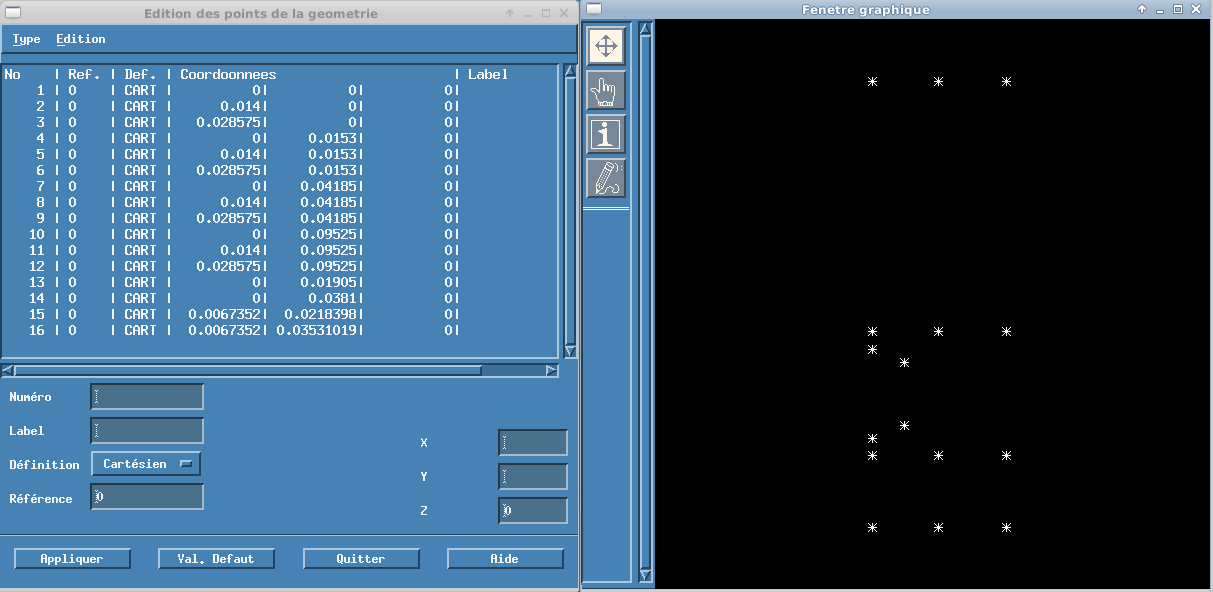Figure 5: Mesh generation, definition of points

Once the points were defined, it was possible to create the lines (Figure 6). A reference number was set for each line, in order to define the limit conditions. The lines on the top were defined as the outlet region –reference 90-, the lateral lines, the bottom and the circle, as walls –reference 50, 51 and 10 respectively-. In this case, there was no inlet of propane, since it was a pool of static liquid with a heating tube.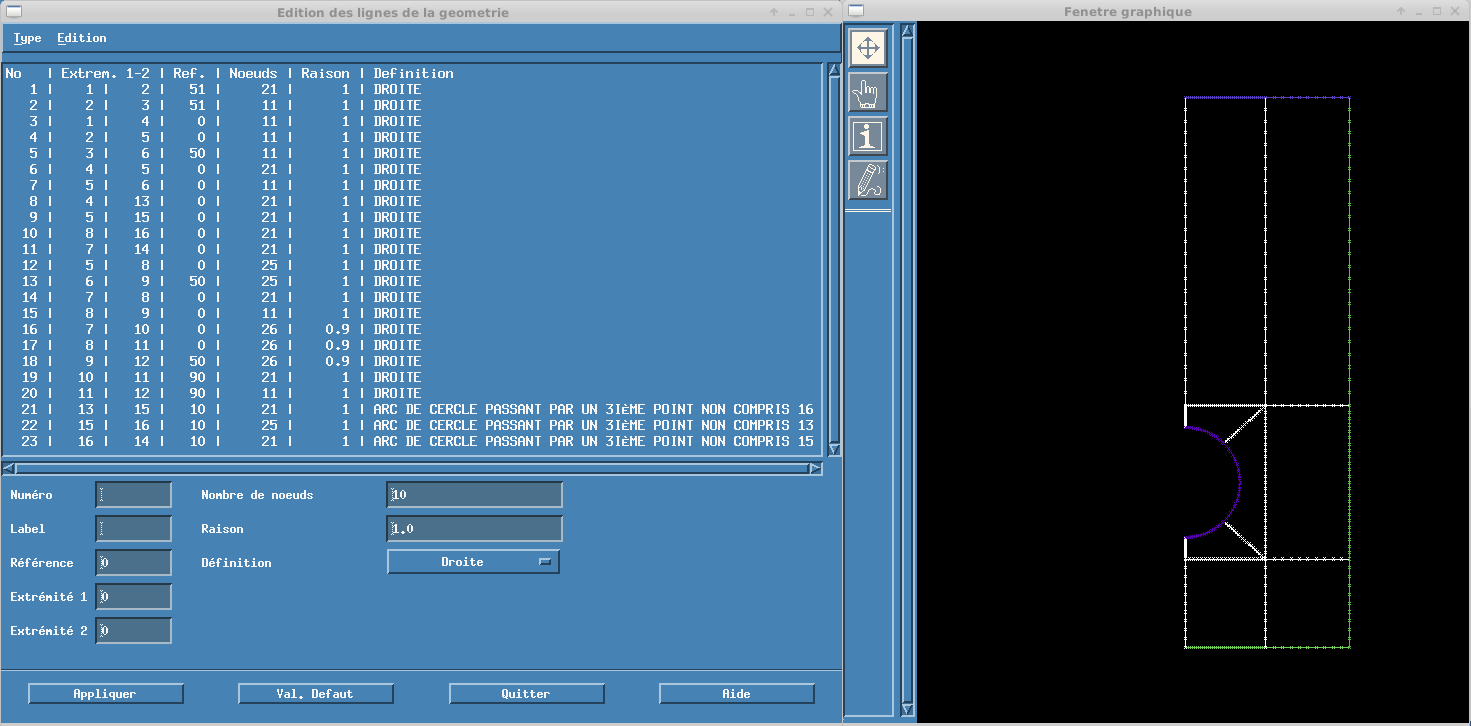Figure 6:  Mesh generation, definition of lines

After that, the meshes in the 3 region delimited by the circle were created using the quadrangulate option. Then, they were joined and regularized to optimize the mesh, removing the discontinuities as displayed in figure 7.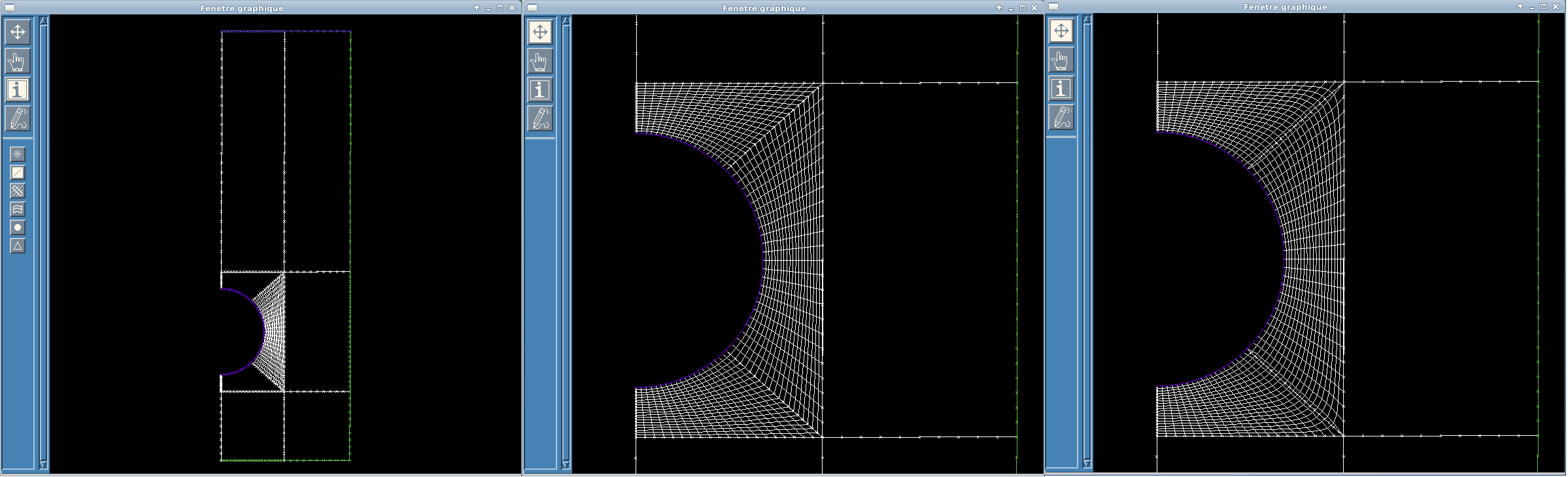Figure 7:  Mesh generation, curved zones

The next step was to create the mesh in the remaining regions, then join them to form only one, and duplicate it to complete the geometry. Once again, the mesh obtained was regularized to have an optimal mesh, particularly in areas with angles.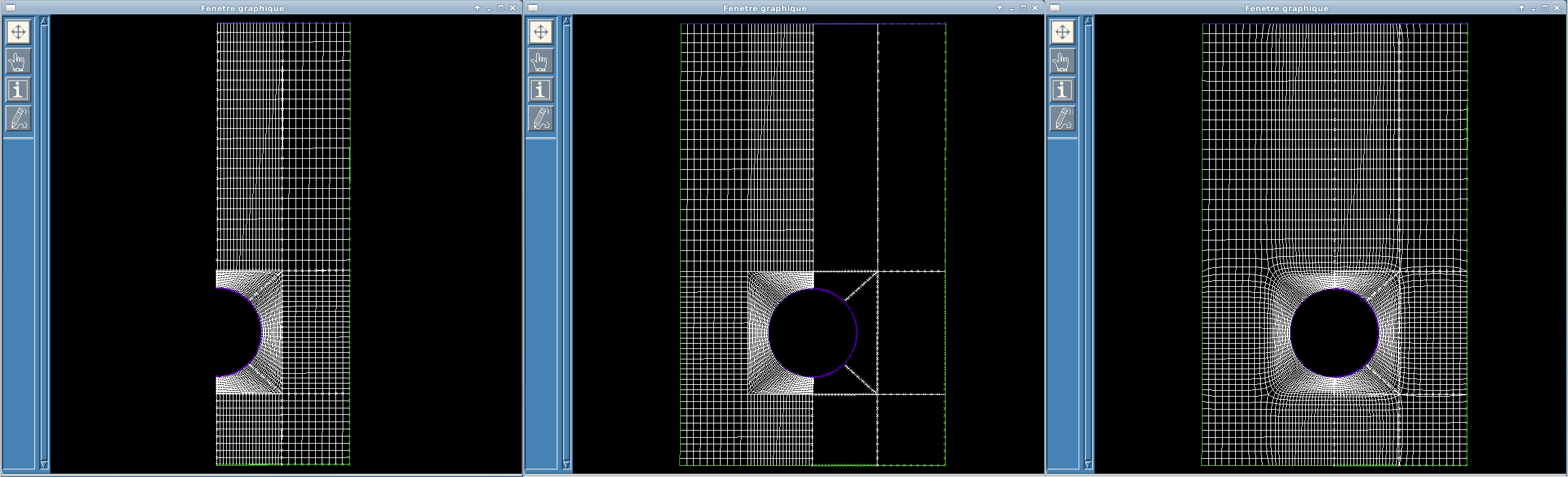Figure 8: Mesh generation, mirrored copy and complete 2D mesh

Finally, a 2D to 3D transformation was performed, since NEPTUNE_CFD only accepts 3D meshes. An elevation of 0.5mm was considered and the symmetry reference was set. The final can be seen in the figure below.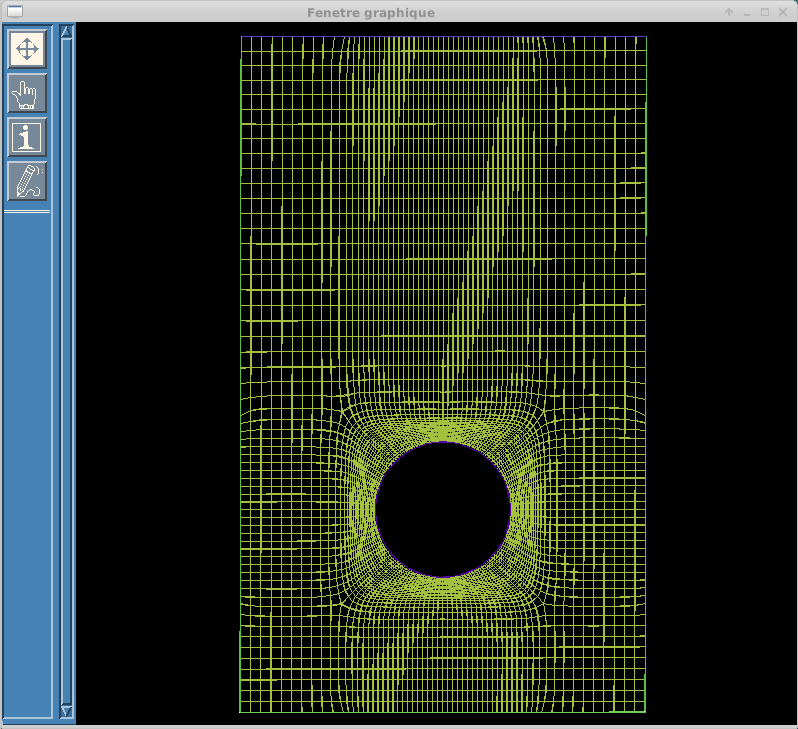Figure 9: Final 3D mesh

Different meshes were used along this study, since it was it was proved that the simulation was mesh-dependant. Additonal information of the mesh used will be presented in the corresponding section.